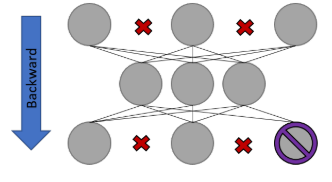# Idiomatic way to selectively zero gradients based on input

I am building a recommender system based on an auto-encoder network, therefore, in order to train the network I have to only calculate the gradients for the weights connected to positive ratings - an input is a collection of ratings and a negative rating means that no rating has been provided.Assuming the input to this networks was [3,-1,2,-1,5], we would get the gradients for those weights removed from the picture above set to zero, so that, they end up being “discarded” when updating the weights, otherwise, the network would learn also the negative ratings and we want to avoid that at any cost because negative ratings are just a placeholder for no rating at all.

I have come up with this model:

``````# -----------------------------------------------------------------------------
# Model definition for AutoRec
# -----------------------------------------------------------------------------

class AutoRecModel(nn.Module):

def __init__(self, D_in, H):
super(AutoRecModel, self).__init__()
self.encoder = nn.Linear(D_in, H)
self.decoder = nn.Linear(H, D_in)
# tie weights
self.decoder.weight.data = self.encoder.weight.data.transpose(0,1)
self.register_buffer('input', torch.zeros(D_in))

def forward(self, x):
self.input = x.clone()
hidden_output = self.encoder(x)
ratings_pred = self.decoder(hidden_output)
return ratings_pred
``````

Then, I coded this training function where some parts were removed for the sake of simplicity:

``````# -----------------------------------------------------------------------------
# Training function for AutoRec
# -----------------------------------------------------------------------------

autorec = arm.AutoRecModel(5, 3)
input = torch.tensor([3,-1,2,-1,5], requires_grad=False)
loss_function = nn.MSELoss()
optimizer = optim.SGD(autorec.parameters(), lr=1e-1)

for i in range(1):

autorec.zero_grad()
pred_y = autorec(input)
loss = loss_function(pred_y, input)
encoder_handle, decoder_handle = add_grad_hooks(zero_encoder_weights, zero_decoder_weights)

loss.backward()
optimizer.step()
encoder_handle.remove()
decoder_handle.remove()
``````

Finally, these are the auxiliary functions:

``````# -----------------------------------------------------------------------------
# Auxiliary functions for AutoRec
# -----------------------------------------------------------------------------

def add_grad_hooks(encoder_hook, decoder_hook):
for name, param in autorec.named_parameters():
if name == "encoder.weight":
encoder_handle = param.register_hook(encoder_hook)
if name == "decoder.weight":
decoder_handle = param.register_hook(decoder_hook)
return encoder_handle, decoder_handle

def zero_weights(grad, network='ENCODER'):
column_index = 0
grad_clone = grad.clone()
for rating in autorec.state_dict().get("input"):
if rating < 0:
if network == 'ENCODER':
grad_clone[:,column_index] = 0
else:
grad_clone[column_index, :] = 0
column_index += 1
print(grad_clone)
return grad_clone

def zero_encoder_weights(grad):
return zero_weights(grad)

def zero_decoder_weights(grad):
return zero_weights(grad, 'DECODER')
``````

Despite the said set up working as expected I would like to know if there is any other way to achieve the same result, something cleaner/more idiomatic for zeroing the grads of some weights based on the input?

Hi
I was using sth similar to your code, my problem is that this makes the training very very slow. Have you experienced the same problem?